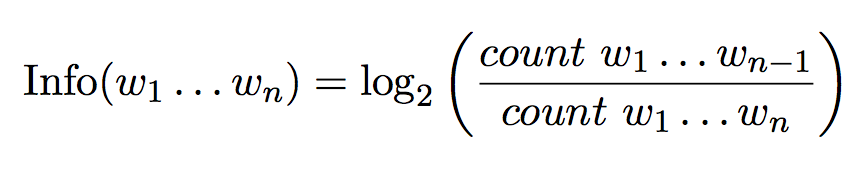# What does NIST information weights refer to?

NIST is a metric used to measure the goodness of translation.

In the paper, Doddington (2002) introduce the notion of "Information weights"

Information weights were computed using N-gram counts over the set of reference translations, according to the following equation:Which Owczarzak (2008) describes as

Here the precision is modified by the information weights Info(w1 . . . wn), computed on the basis of the frequency of a given n-gram in relation to the frequency of a ‘parent’ (n-1)-gram, in accordance with the equation (2.6)

There's an implementation of the NIST score on https://github.com/moses-smt/mosesdecoder/blob/master/scripts/generic/mteval-v13a.pl

But we can't seem to figure out how should the information weights be computed on https://github.com/nltk/nltk/pull/1840

Does anyone know the details of how this information weights work?

I've tried looking at the mteval-v13a.pl script but I'm not understanding how it works and relates to the formula above.